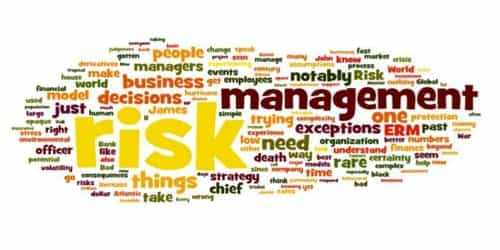# Quantitative techniques of Environmental Forecasting

Quantitative techniques of Environmental Forecasting

In today’s business world rapid changes are too frequent. It would be crucial for managers to invent new ways of surviving in the ever-changing business environment. They would have to build up the capacity of a firm to face the changes and adapting themselves to changes. To prepare for such ongoing eventualities, managers will have to prepare themselves for really understanding the remote and the immediate environments of business and mechanisms of changes that affect their industry or firm. All forecasting techniques can be classified as either qualitative or quantitative. Quantitative techniques are based primarily on the analysis of data and the use of statistical techniques.

Quantitative Forecasting Techniques:

• Time-Series Analysis:

This technique forecasts future demand based on what has happened in the past. The basic idea of time-series analysis is to fit .a trend ‘line to past data and then to extrapolate this trend line into the future.

Sophisticated mathematical procedures are used to derive this trend line and to identify and seasonal or cyclical fluctuations. Usually, a computer program is used to do the calculations required by a time-series analysis.

One advantage of this technique is that it is based on something other than opinion. This method works best when a significant amount of historical data is available and when the environmental forces are relatively stable. The disadvantage is that the future may not be like the past.

• Regression Modeling:

Regression modeling is a mathematical forecasting technique in which an equation with one or more input variables is derived to predict another variable. The variable being predicted is called the dependent variable. The input variables used to predict the dependent variable are called independent variables.

The general idea of regression modeling is not determining how changes in the independent variables affect the dependent variable. Once the mathematical relationship between the independent variables and the dependent variable has been I determined, future values for the dependent Variable can be forecast based on known or predicted values of the independent variables.

The mathematical calculations required to derive the equation are extremely complex and almost always require the use of a computer. Regression modeling is relatively complex and expensive.

• Econometric Modeling:

Econometric modeling is one of the most sophisticated .methods of forecasting. In general, econometric models attempt to mathematically model an entire economy. Most econometric models are based on numerous regression equations that attempt to describe the relationships between the different sectors of the economy.

Very few organizations are capable of developing their own econometric models. Those organizations that do use econometric models usually hire the services of consulting groups or company that specializes in econometric modeling. This method is very expensive and complex and is, therefore, primarily used only by very large organizations.